# DAV Class 8 Maths Chapter 8 Worksheet 3 Solutions

The DAV Class 8 Maths Solutions and DAV Class 8 Maths Chapter 8 Worksheet 3 Solutions of Polynomials offer comprehensive answers to textbook questions.

## DAV Class 8 Maths Ch 8 Worksheet 3 Solutions

Question 1.
Using factor method, divide the following polynomials by a binomial:

(i) x2 + 3x + 2 by x + 1
Solution:
x2 + 3x + 2 ÷ x + 1
$$\frac{x^2+3 x+2}{x+1}$$ = $$\frac{x^2+2 x+x+2}{x+1}$$
= $$\frac{x(x+2)+1(x+2)}{x+1}$$
= $$\frac{(x+1)(x+2)}{x+1}$$
= x + 2
Hence the quotient is (x + 2)

(ii) x2 – 7x – 18 by x – 9
Solution:
x2 – 7x – 18 ÷ x – 9
$$\frac{x^2-7 x-18}{x-9}$$ = $$\frac{x^2-9 x+2 x-18}{x-9}$$
= $$\frac{x(x-9)+2(x-9)}{x-9}$$
= $$\frac{(x+2)(x-9)}{x-9}$$
= x + 2
Hence the quotient is (x + 2)

(iii) p2 + 6p + 8 by p + 2
Solution:
p2 + 6p + 8 ÷ p + 2
$$\frac{p^2+6 p+8}{p+2}$$ = $$\frac{p^2+4 p+2 p+8}{p+2}$$
= $$\frac{p(p+4)+2(p+4)}{p+2}$$
= $$\frac{(p+2)(p+4)}{(p+2)}$$
= (p + 4)
Hence the quotient is (p + 4)

(iv) p2 – p – 42 by p + 6
Solution:
p2 – p – 42 ÷ p + 6
$$\frac{p^2-p-42}{p+6}$$ = $$\frac{p^2-7 p+6 p-42}{p+6}$$
= $$\frac{p(p-7)+6(p-7)}{p+6}$$
= $$\frac{(p+6)(p-7)}{p+6}$$
= (p – 7)
Hence the quotient is (p – 7).(v) y2 + 12y + 35 by y + 7
Solution:
y2 + 12y + 35 ÷ y + 7
$$\frac{y^2+12 y+35}{y+7}$$ = $$\frac{y^2+7 y+5 y+35}{y+7}$$
= $$\frac{y(y+7)+5(y+7)}{y+7}$$
= $$\frac{(y+7)(y+5)}{(y+7)}$$
= y + 5
Hence the quotient is (y + 5).

(vi) z2 – 10z + 16 by z – 2
Solution:
z2 – 10z + 16 ÷ z – 2
$$\frac{z^2-10 z+16}{z-2}$$ = $$\frac{z^2-8 z-2 z+16}{z-2}$$
= $$\frac{z(z-8)-2(z-8)}{z-2}$$
= $$\frac{(z-8)(z-2)}{(z-2)}$$
= z – 8
Hence the quotient is (z – 8).

(vii) x4 + 3x2 – 10 by x2 + 5 (Hint: put x2 = y)
Solution:
x4 + 3x2 – 10 ÷ x2 + 5
$$\frac{x^4+3 x^2-10}{x^2+5}$$ = $$\frac{y^2+3 y-10}{y+5}$$
= $$\frac{y^2+5 y-2 y-10}{y+5}$$
= $$\frac{y(y+5)-2(y+5)}{(y+5)}$$
= $$\frac{(y+5)(y-2)}{(y+5)}$$
= y – 2
= x2 – 2
Hence the quotient is (x2 – 2).

(viii) x2 – 7x + 12 by x – 4
Solution:
x2 – 7x + 12 ÷ x – 4
$$\frac{x^2-7 x+12}{x-4}$$ = $$\frac{x^2-4 x-3 x+12}{x-4}$$
= $$\frac{x(x-4)-3(x-4)}{x-4}$$
= $$\frac{(x-4)(x-3)}{x-4}$$
= (x – 3)
Hence the quotient is (x – 3).Question 2.
Using long division method, divide the following polynomials by a binomial and check your answer.

(i) 4p3 – 4p2 + 6p – $$\frac{5}{2}$$ by 2p – 1
(ii) x – 6 + 15x2 by 2 + 3x
(iii) 4x3 – 37x2 + 52x – 15 by 4x – 5
(iv) y3 – 8 by y – 2
(v) 29z – 6z2 – 28 by 3z – 4
(vi) p4 + p3 – p2 + 1 by p – 1
(vii) 12z3 + 4z + 3z2 + 1 by 4z + 1
(viii) 8x2 – 2 – 3x + 12x3 by 4x2 – 1
Solution:
(i) 4p3 – 4p2 + 6p – $$\frac{5}{2}$$ ÷ 2p – 1Check:
Dividend = Divisor × Quotient + Remainder
4p3 – 4p2 + 6p – $$\frac{5}{2}$$ = (2p – 1) × (2P2 – p + $$\frac{5}{2}$$) + o
= 2p (2p2 – p + $$\frac{5}{2}$$) – 1 (2P2 – p + $$\frac{5}{2}$$)
= 4p3 – 2p2 + 5p – 2p2 + p – $$\frac{5}{2}$$
= 4p3 – 4p2 + 6p – $$\frac{5}{2}$$
⇒ L.H.S. = R.H.S.
Hence verified.

(ii) Let us write dividend and divisor in standard form.
Dividend = 15x2 + x – 6
and Divisor = 3x + 2Check:
Dividend = Divisor × Quotient + Remainder
15x2 + x – 6 = (3x + 2) × (5x – 3) + 0
= 3x (5x – 3) + 2 (5x – 3)
= 15x2 – 9x + 10x – 6
= 15x2 + x – 6
⇒ L.H.S. = R.H.S.
Hence verified.(iii) 4x3 – 37x2 + 52x – 15 ÷ 4x – 5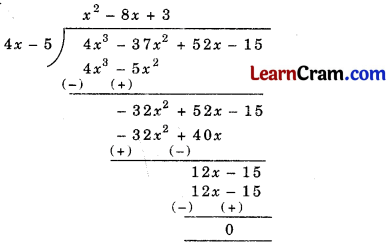Check:
Dividend = Divisor × Quotient + Remainder
4x3 – 37x2 + 52x – 15 = (4x – 5) x (x2 – 8x + 3) + 0
= 4x (x2 – 8x + 3) – 5 (x2 – 8x + 3)
= 4x3 – 32x2 + 12x – 5x2 + 40x – 15
= 4x3 – 37x2 + 52x – 15
⇒ L.H.S. = R.H.S.
Hence Verified.

(iv) y3 – 8 ÷ y – 2Check:
Dividend = Divisor × Quotient + Remainder
L.H.S. = y3 – 8
R.H.S. = (y – 2) (y2 + 2y + 4) + 0
= y (y2 + 2y + 4) – 2 (y2 + 2y + 4)
= 9 + 2y2 + 4y – 2y2 – 4y – 8
= y3 – 8
⇒ L.H.S. = R.H.S.
Hence Verified.(v) Let us write the dividend in standard form.
We have 29 – 6z2 – 28 = – 6z2 + 29z – 28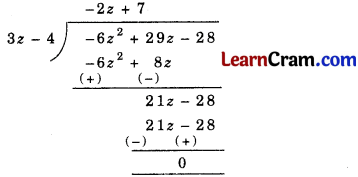Check:
Dividend = Divisor × Quotient + Remainder
– 6z2 + 29z – 28 = (3z – 4) × (- 2z + 7) + 0
= 3z (- 2z + 7) – 4(- 2z + 7)
= – 6z2 + 21z + 8z – 28
= – 6z2 + 29z – 28
⇒ L.H.S. = R.H.S.
Hence verified.

(vi) p4 + p3 – p2 + 1 ÷ p – 1Quotient = p3 + 2p2 + p + 1
Remainder = 2

Check:
Dividend = Divisor × Quotient + Remainder
p4 + p3 – p2 + 1 = (p – 1) (p3 + 2p2 +p + 1)
= p (p3 + 2p2 + p + 1) – 1 (p3 + 2p2 + p + 1)
= p4 + 2p3 + p2 + p – p3 – 2p2 – p – 1
= p4 + p3 – p2 – 1
∴ L.H.S. = R.H.S.
Hence verified.(vii) Let us write the dividend in standard form:
Dividend = 12z3 + 3z2 + 4z + 1Check:
Dividend = Divisor × Quotient + Remainder
12z3 + 3z2 + 4z + 1 = (4z + 1) (3z2 + 1) + 0
= 4z (3z2 + 1) + 1(3z2 + 1)
= 12z3 + 4z + 3z2 + 1
= 12z3 + 3z2 + 4z + 1
L.H.S. = R.H.S.
Hence verified.

(viii) Let us write the dividend in standard form:
Dividend = 12x3 + 8x2 – 3x – 2Check:
Dividend = Divisor × Quotient + Remainder
12x3 + 8x2 – 3x – 2 = (4x2 – 1) × (3x + 2) + 0
= 4x2 (3x + 2) – 1 (3x + 2)
= 12x3 + 8x2 – 3x – 2.Question 3.
Using long division method, check whether the second polynomial is the factor of the first polynomial.
(i) x4 – 16 ; x + 2
(ii) z4 – z3 + 3z2 – 2z + 2 ; z2 + 2
(iii) x3 – 4x2 – 3x + 5 ; x – 3
(iv) 4p3 – 12p2 – 37p – 15 ; 2p + 1
(v) 4q3 – 6q2 – 4q + 3 ; 2q – 1
(vi) 20x2 – 32x – 16 ; 5x + 2
(vii) 12a2 – 7a – 2 ; 4a – 1
(viii) 6x3 + 19x2 + 13x + (- 3) ; 2x + 3
Solution:
(i) x4 – 16 ; x + 2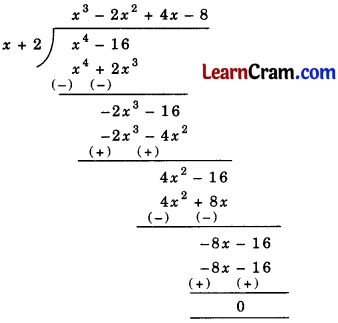Here, remainder = 0
Hence (x + 2) is a factor of x4 – 16.

(ii) z4 – z3 + 3z2 – 2z + 2 ; z2 + 2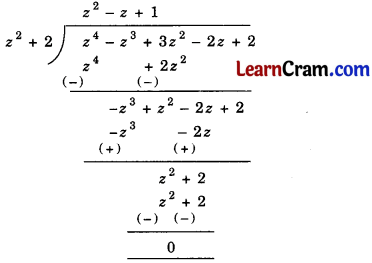Here, the remainder = 0
Hence z2 + 2 is a factor of z4 – z3 + 3z2 – 2z + 2.

(iii) x3 – 4x2 – 3x + 5 ; x – 3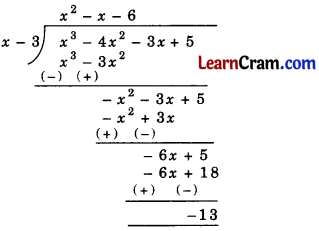As remainder = – 13 which is not equal to 0.
So, x – 3 is not a factor of x3 – 4x2 – 3x + 5.

(iv) 4p3 – 12p2 – 37p – 15 ; 2p + 1Here, remainder = 0
Thus, (2p + 1) is a factor of 4p3 – 12p2 – 37p – 15.(v) 4q3 – 6q2 – 4q + 3 ; 2q – 1Here, remainder = 0
Thus, (2q – 1) is a factor of 4q3 – 6q2 – 4q + 3.

(vi) 20x2 – 32x – 16 ; 5x + 2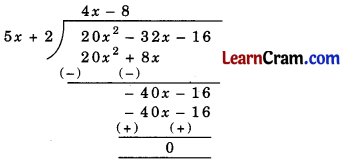Here, remainder = 0
Thus, (5x + 2) is a factor of 20x2 – 32x – 16.

(vii) 12a2 – 7a – 2 ; 4a – 1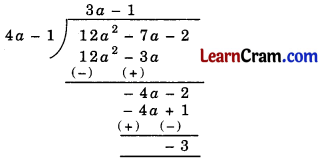As remainder = – 3 which is not equal to 0.
So 4a – 1 is not a factor of 12a2 – 7a – 2.

(viii) 6x3 + 19x2 + 13x + (- 3) ; 2x + 3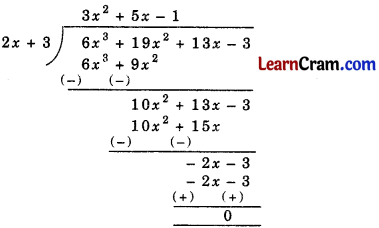Here, remainder = 0
Thus, (2x + 3) is a factor of 6x3 + 19x2 + 13x – 8.### DAV Class 8 Maths Chapter 8 Value Based Questions

Question 1.
Mr. Lalit donated (x2 + 12x + 35) for the education of (x + 7) children.
(i) Find the amount received by each child, if each child got the equal amount.
(ii) Why it is necessary to educate children? Give one reason.
Solution:
(i) Total donation = (x2 + 12x + 35)
= [x2 + (7 + 5) x + 7 × 5]
= [x2 + 7x + 5x + 7 × 5]
= [x (x + 7) + 5 (x + 7)]
= (x + 7) (x + 5)
Number of children = (x + 7)
Amount received by each child = $$\frac{(x+7)(x+5)}{(x+7)}$$ = (x + 5)

(ii) Children is the future asset of the nation as they have to represent the nation.
So, it is necessary to educate children.Question 2.
Every morning Abhay goes for Jogging in a park.
(i) Find the time taken by him to cover a distance of (2t4 + 3t3 – 2t2 – 9t – 12) km at the speed of (t2 – 3) km/hr.
(ii) Write the importance of physical exercises like jogging, walking, etc.
Solution:
(i) Distance = (2t4 + 3t3 – 2t2 – 9t – 12) km
Speed = (t2 – 3) km/hr
Time = $$\frac{\text { Distance }}{\text { Speed }}$$
= $$\frac{\left(2 t^4+3 t^3-2 t^2-9 t-12\right) \mathrm{km}}{\left(t^2-3\right) \mathrm{km} / \mathrm{hr}}$$= (2t2 + 3t + 4) hr

(ii) Physical exercises keeps the body fit and sound.